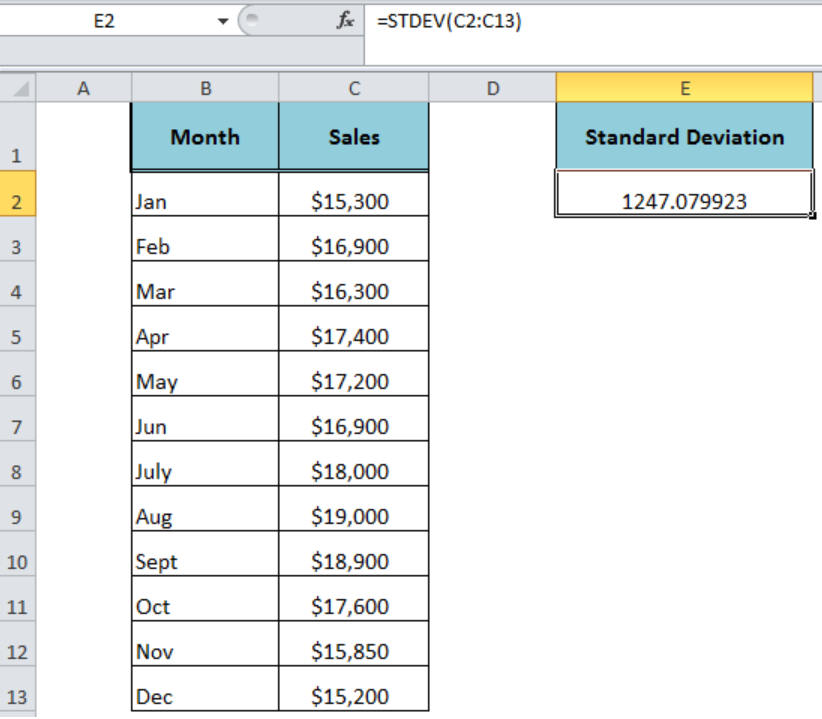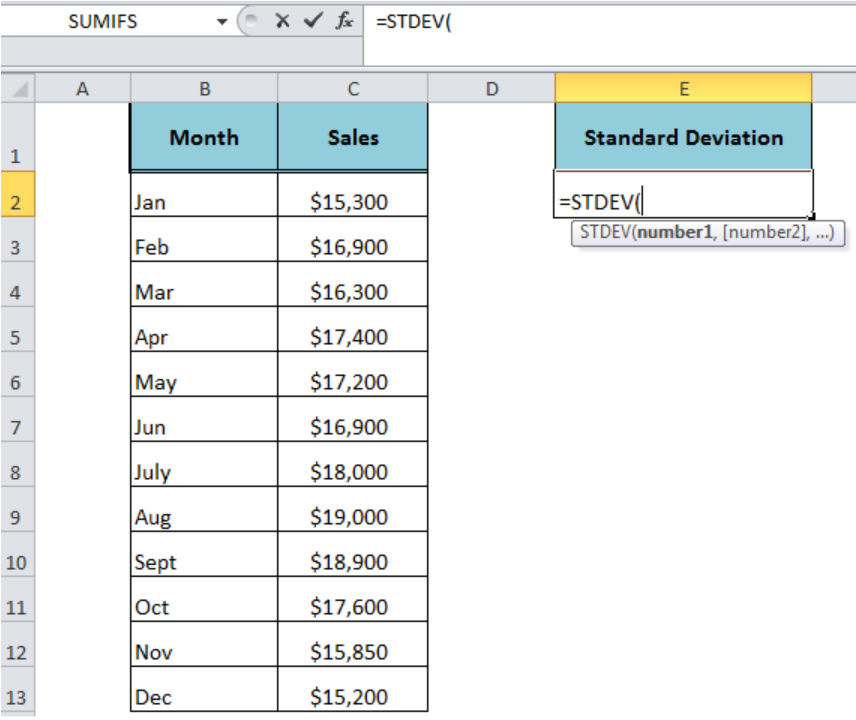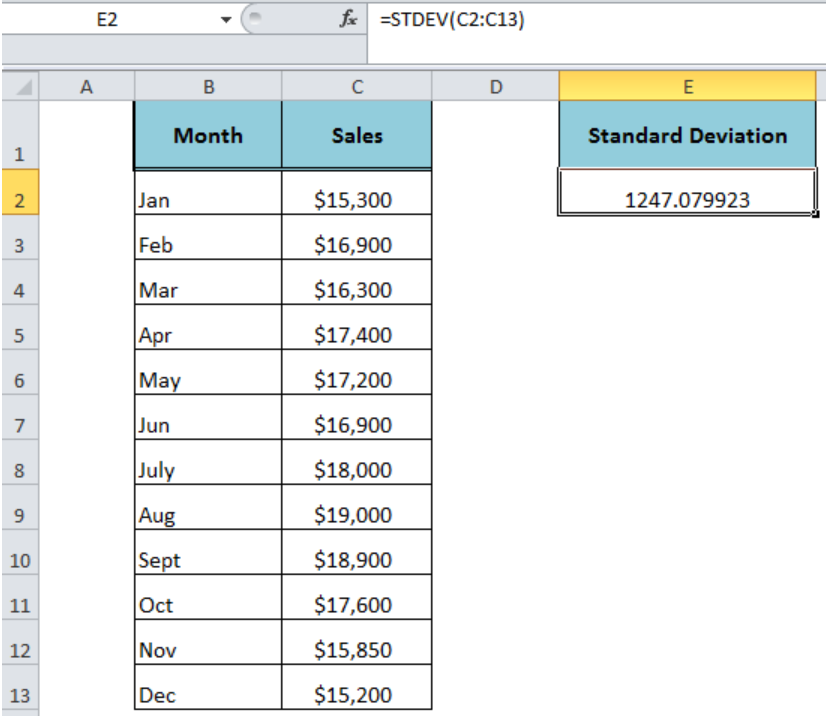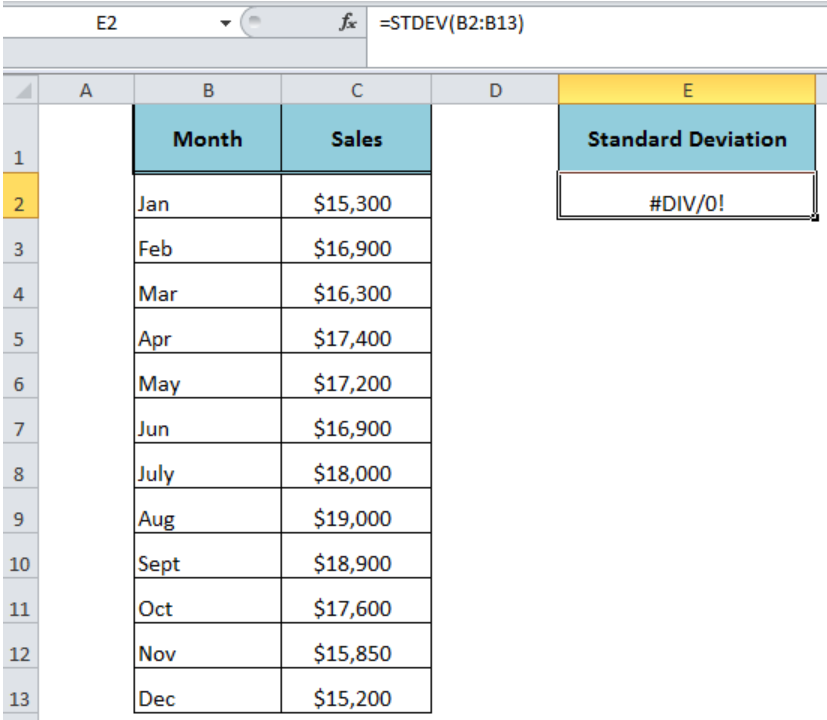Get instant live expert help with Excel or Google Sheets“My Excelchat expert helped me in less than 20 minutes, saving me what would have been 5 hours of work!”

#### Post your problem and you’ll get Expert help in seconds.

Your message must be at least 40 characters
Our professional Expert are available now. Your privacy is guaranteed.

# Excel STDEV Function

The standard deviation is the measure of the variance in a set of numbers compared to the mean or average of the numbers. The Excel STDEV function calculates the standard deviation of a set of numbers that represents a sample.Figure 1. How to Use the Excel STDEV Function

## Syntax

The syntax for the Excel STDEV function is;

`=STDEV(number1,[number2],...)`

This function can have arguments between 1 and 255 maximum.

• number1 – It is required and the first argument of sample number
• number2,… – These are optional and additional arguments of sample numbers between 2 and 255.

The Excel STDEV function supports arguments that can be entered as numbers, arrays, ranges or cell references that may contain numeric, text, logical values, text representations of numbers and empty cells. It evaluates only numeric values in the calculation of standard deviation, ignoring the text, logical values (TRUE and FALSE), text representations of numbers and empty cells in ranges, arrays or cell references of a sample set of values. However, the logical and text representations of numbers supplied directly as the argument in function are evaluated as numbers.Figure 2. The Syntax For the Excel STDEV Function

## Example

Suppose we have a sample dataset of monthly sales and we need to calculate the standard deviation based on this sample dataset. This estimate basically tells us how much monthly sales figures deviate from the average monthly sales of the sample. Following formula will be used to calculate a standard deviation of this sample;

`=STDEV(C2: C13)`Figure 3. Calculating the Standard Deviation of Sample

## Usage Notes

• The Excel STDEV function has been replaced by the STDEV.S function in Excel 2010 and later versions. It is now available for compatibility with Excel 2007 and earlier.
• It generates #DIV/0! error if all supplied arguments contain text values.
• If a text value is entered directly as an argument, it generates #VALUE! error.Figure 4. Error Value of the STDEV Function

## Instant Connection to an Expert through our Excelchat Service:

Most of the time, the problem you will need to solve will be more complex than a simple application of a formula or function. If you want to save hours of research and frustration, try our live Excelchat service! Our Excel Experts are available 24/7 to answer any Excel question you may have. We guarantee a connection within 30 seconds and a customized solution within 20 minutes.

### Did this post not answer your question? Get a solution from connecting with the expert.Another blog reader asked this question today on Excelchat:## Subscribe to Excelchat.coAnother blog reader asked this question today on Excelchat: